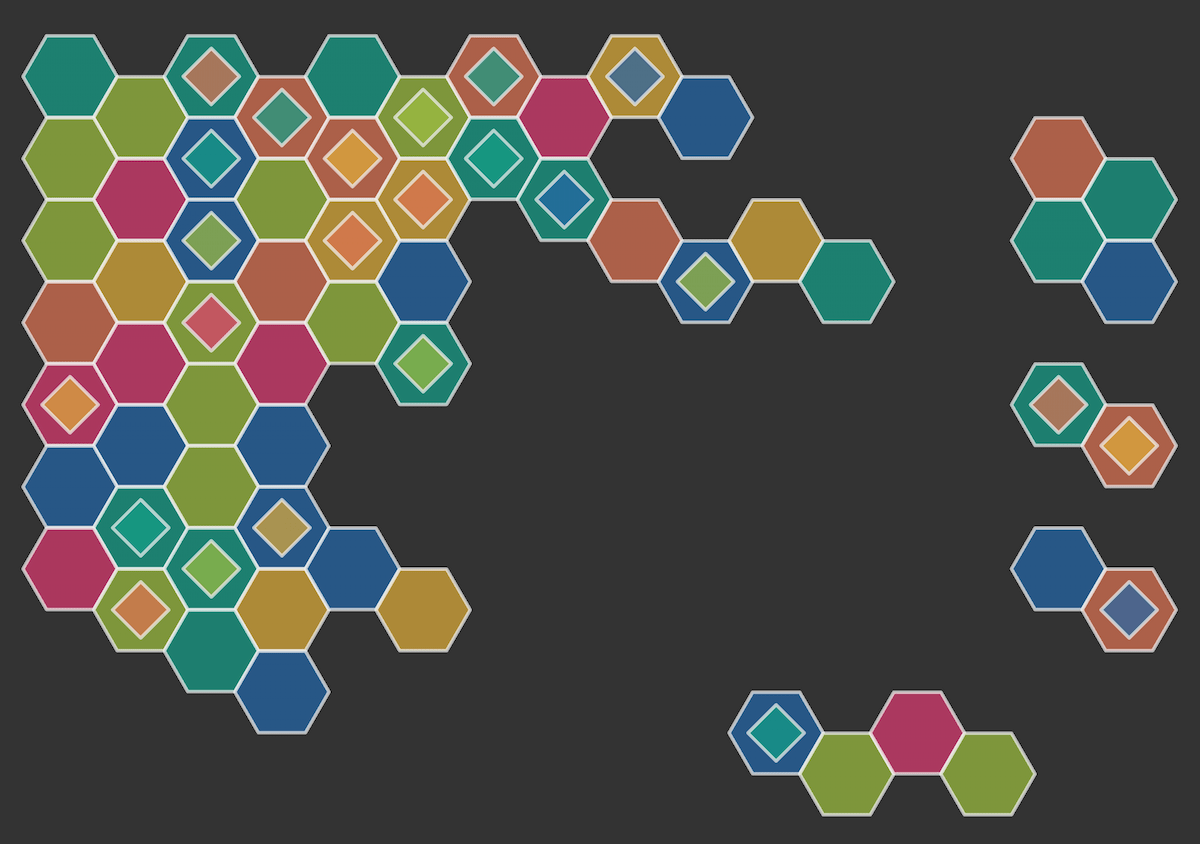# How to draw any regular shape with just one JavaScript function

Ok alright, I know I used a clickbait title, but bear with me. I want to share a function I've been using for ages. I originally wrote it to draw a hexagon – hexagons are cool, lots of hexagons are better, and tessellated hexagons are the best. So I wrote a function to draw one, which I could then repeat.

As I was doing so, I started modifying the hexagon to enable drawing a number of shapes that are based on just a couple of parameters. Let's begin with what I did with the hexagon and we'll take it from there.

## Drawing a hexagon with JavaScript

Hexagons have six equal sides. If we imagine our starting point as the center of the hexagon, we can move around this point six times, joining each point as we go to make the sides.

Let's start off by creating a `<canvas>` with a `2d` drawing context. We'll fix the size of the canvas to 400 x 200 pixels for this example and set the center point as `(200, 100)`.

html

``````<canvas></canvas>
``````

js

``````const canvas = document.querySelector("canvas");
canvas.width = 400;
canvas.height = 200;

const ctx = canvas.getContext("2d");
const cx = 200;
const cy = 100;
``````

Now we need to figure out the x (horizontal) and y (vertical) position of points around the center, which when joined with a line, will make six equal sides. For this, we use the measurement from the center to the point (we'll call this the radius) and the angle of direction from the center.

As there are 360 degrees in a full rotation and six points we want to create, we can divide 360/6 and know we'll make a point every 60 degrees. However, there's a tiny caveat to this – JavaScript works with `radians` rather than `degrees`. One thing I always remember is that the value `pi` in `radians` is 180 degrees, or half a circle. So `(Math.PI*2)/6` would give us each rotation in `radians` or even simpler `Math.PI/3`.

Next we need to add a bit of trigonometry to find the x and y position of each point. For the x position, we can use the sum radius multiplied by cos(angle) and for the y position radius multiplied by sin(angle). Let's put it all together, adding to our JavaScript code above:

js

``````// set the radius of the hexagon

// move the canvas to the center position
ctx.translate(cx, cy);

for (let i = 0; i < 6; i++) {
// calculate the rotation
const rotation = (Math.PI / 3) * i;

// for the first point move to
if (i === 0) {
} else {
// for the rest draw a line
}
}

// close path and stroke it
ctx.closePath();
ctx.stroke();
``````

## Drawing a shape with any number of sides

Let's say we wanted to draw a triangle, a square, or an octagon. All we would need to modify in the above function, used to draw the hexagon, is the number of times we draw lines in our `for` loop and the angle for each point.

Let's turn this into a function that takes the center point, the radius, and number of sides as parameters:

js

``````function drawShape(x, y, r, sides) {
// move the canvas to the center position
ctx.translate(x, y);

for (let i = 0; i < sides; i++) {
// calculate the rotation
const rotation = ((Math.PI * 2) / sides) * i;

// for the first point move to
if (i === 0) {
ctx.moveTo(r * Math.cos(rotation), r * Math.sin(rotation));
} else {
// for the rest draw a line
ctx.lineTo(r * Math.cos(rotation), r * Math.sin(rotation));
}
}

// close path and stroke it
ctx.closePath();
ctx.stroke();

// reset the translate position
ctx.resetTransform();
}
``````

Now we can draw different shapes by adjusting the `sides` parameter:

js

``````drawShape(100, 100, 50, 3);
drawShape(225, 100, 50, 7);
drawShape(350, 100, 50, 4);
``````

## Summary

This was a little introduction to the `<canvas>` element for drawing on a web page and a few of the methods you can use to draw shapes. If you want to dive deeper into how all the pieces work, here's a recap of what we used:

To calculate the position of each point, we used a little bit of maths and trigonometry:

To get some more inspiration for what you can do with the `<canvas>` element, check out the Canvas tutorial that starts off with the basics and then covers more advanced topics like animation and pixel manipulation.

There are plenty of ways you can expand on this basic shape function. I like to include an inner radius, so you can create diamonds and stars. I've also experimented a little with curves instead of straight lines - feel free to experiment for yourself. Or try some tessellation, which is always fun!Let me know if you try out this function and if you like it as much as I do. As always, feel free to leave any feedback on the GitHub discussion or join us for a chat in the MDN Web Docs Discord server.

## Stay Informed with MDN

Get the MDN newsletter and never miss an update on the latest web development trends, tips, and best practices.